# Algebra 1 : How to find mode

## Example Questions

### Example Question #41 : How To Find Mode

What is the mode of the following set of numbers?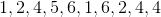Explanation:

We define mode as the number that appears most often in a set of numbers.Examining the set above, we see thatappears the most times specifically, it appears three times. The most any other number appears in the set is two times and therefore the mode of the set is### Example Question #42 : How To Find Mode

What is the mode of the following set of numbers?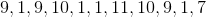Explanation:

We define mode as the number that appears most often in a set of numbers.Examining the set above, we see thatappears four times in the data set. The most any other number appears in the set is the numberwhich appears only three times thereforeis the mode of the set.

### Example Question #42 : How To Find Mode

What is the mode of the following set of numbers?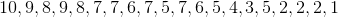Explanation:

We define mode as the number that appears most often in a set of numbers.If we arrange the set in ascending order it is easier to identify the mode.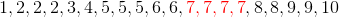Examining the set above, we see thatappears the most times and is therefore the mode of the set.

### Example Question #62 : Basic Statistics

Consider the following set of numbers:Find the mode of the set.Explanation:

The mode of the set is the most repeated number in the set.

First organize the set in order from smallest to largest.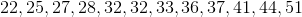From here we can see more clearly when there is a repeated number.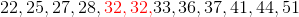In this case that number isbecause all other number only occur once.

### Example Question #62 : Basic Statistics

Consider the following set of numbers: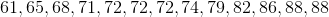Find the mode of the set.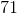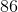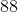Explanation:

The mode of the set is the most repeated number in the set. In this case there is number being repeated once and another being repeated twice.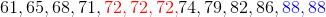Therefore the answer will be the number that is being repeated twice,.

This number can be seen in red.

### Example Question #62 : Basic Statistics

Consider the following set: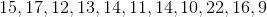Find the mode of the set.Explanation:

The mode of the set is the number that occurs the most times in the set.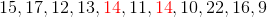In this case that number issince all other numbers are only seen once in the data set.

### Example Question #401 : Statistics And Probability

What is the mode of the following data set?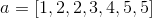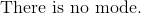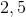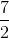Explanation:

The dataset is already in chronological order.  The meaning of mode is the value that occurs most often in a data set.  There can be more than one mode.

There are two of each forandin the data set.

Therefore, the answer is:### Example Question #45 : How To Find Mode

In the following data set, which value represents the mode?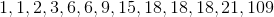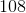Explanation:

The mode describes the number in the data set that occurs most frequently, i.e. which number appears the most number of times in the list. In this data set,occurs three times, which is more than any other value, therefore it is the mode.

### Example Question #49 : How To Find Mode

What is the mode of the following set of numbers?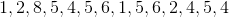Explanation:

We define mode as the number that appears most often in a set of numbers. Examining the set above, we see thatappears the most times and is therefore the mode of the set.

### Example Question #50 : How To Find Mode

What is the mode of the following set of numbers?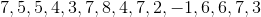We define mode as the number that appears most often in a set of numbers. Examining the set above, we see thatappears the most times and is therefore the mode of the set.